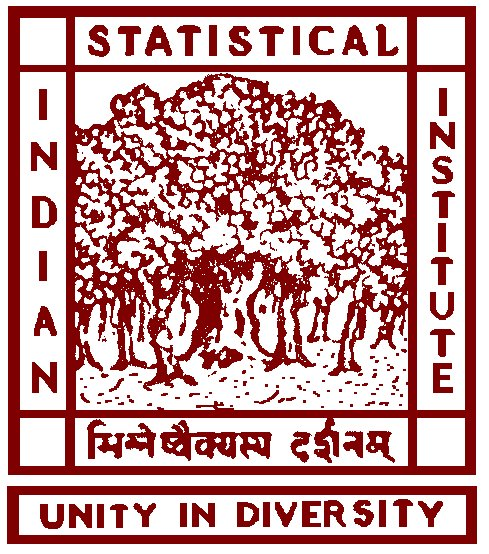# INDIAN STATISTICAL INSTITUTE

### 203 B. To Road, Kolkata 700 108# Probability Theory III

## Academic Year 2020 - 2021: Semester I

Instructor: Antar Bandyopadhyay
Email: antar (at) isid (dot) ac (dot) in
Office:

• Room # 208 on the First Floor of the Faculty Block (when in Delhi); and
• Room # 3.4 on the Third Floor of the A. N. Kolmogorov Bhavan (when in Kolkata).

Teaching Assistant (TA): Partha Pratim Ghosh
Email: p (dot) pratim (dot) 10 (dot) 93 (at) gmail (dot) com

Weekly Class Time:

• Lecture Hours: Monday, Wednesday and Thursday 11:30 AM - 12:20 PM;
• Contact Hour/Tutorial: Friday 02:00 PM - 02:50 PM.

Lecture Hall: Online Google Classroom and online class via Zoom Meetings

Course Duration (including examinations): September 14 - January 08, 2021
[Total of 17 Weeks = 14 Weeks of Classes + 1 week of Study Leave + 2 weeks of Final Examination Weeks].

Midterm Examination: WAIVED due to the pandemic!

Final Examination:
Date: January 04, 2021
Time: 02:30 PM - 06:30 PM (including the time for uploading the scanned answer sheets)
Venue: Online Google Classroom and Zoom Meeting

Course Outline:

• Revision of the concept of cumulative distribution function, inverse distribution function, the Fundamental Theorem of Probability. [1 week]
• General definition of Expectation. Properties of expectation. Limit theorems: Monotone Convergence Theorem (MCT), Fatou's Lemma, Dominated Convergence Theorem (DCT). Scheffe's Theorem. Change of variable formula. Expectation for discrete and continuous random variables. Law of unconscious Statistician. [4 weeks]
• Markov, Chebyshev, Cauchy-Schwartz, Hölder, Minkowsky and Jensen inequalities. [1 week]
• First and Second Borel-Cantelli Lemmas. Various modes of convergence and their interrelations. WLLN and SLLN. Kolmogorov Maximal inequality. Lévy's convergence Theorem. Kolmogorov Three Series Theorem (proof of the sufficient part). Fundamental Theorem of Statistics (Glivenko-Cantelli Lemma). [2 weeks]
• Weak convergence, definition. Weak convergence through convergence of probability mass functions and convergence of probability density functions. Representation theorem, Portmanteau Theorem. Slutsky's Theorem. Hally-Bray Theorem, tightness. [2 weeks]
• Characteristic functions, definition, examples. Moment expansion, characteristics function for Normal distribution. Inversion formula, uniqueness theorem, density formula. Characteristic functions and weak convergence, Lévy's Continuity Theorem (statement only). [3 weeks]
• I.I.D. Central Limit Theorem (CLT). Lyaponov's CLT (statement only). Proof of the necessity part of the Kolmogorov Three Series Theorem. δ-method. Multivariate CLT, Cramer-Wald device. [1 week]

References:

• W. Feller: Introduction to the Theory of Probability and its Applications: Volume I & II.
• K. L. Chung: Elementary Probability Theory.
• P. G. Hoel, S. C. Port and C. J. Stone: Basic Probability Theory.
• S. M. Ross: A First Course in Probability.

Grading Policy:

• Assignments: 50% of the total credit.
• Final Exam: 50% of the total credit

Assignment Policies:

• There will be a total of 14 sets of homework assignments each carrying a total of 10 points. The average of all the 14 assignment scores will be taken for the final grading.
• The assignments will be given every Friday, starting from September 18, 2020. Each assignment will be due on the following Friday. For example the first assignment is due on Friday, September 25, 2020.
• Each assignment will be based on the course materials which will be covered in the class in the week of the assignment.
• Late submission of an assignment will NOT BE ACCEPTED.
• Graded assignments will be returned individually.

Exam Policies:

• The Final Examination will be open notes examinations. That means, students will be allowed to bring his/her own hand written notes, study materials, list of theorems etc. But no printed or photocopied materials will be allowed.
• Any unfair means used by any students in the examinations will be dealt with the strictest possible measures, as per the Institute rules. In particular, if any student is found to be using any kind of unfair practice during any of the examinations (including the quizzes) then he/she will be awarded ZERO in that examination.

Regrading Policy:

• Regrading of the assignments or exams will only be undertaken in cases where, you believe there has been a genuine error or misunderstanding. Please note that our primary aim in grading is consistency, so that all students are treated the same; for this reason, we will not adjust the score of one student on an issue of partial credit, unless the score allocated clearly deviates from the grading policy we adopted for that problem.
• If you wish to request a regrading of a homework or exam, you must return it to the instructor with a written note on a separate piece of paper explaining the problem.
• The entire assignment or the exam may be regraded, so be sure to check the solutions to ensure that your overall score will go up after regrading.
• All such requests must be received within one week from the date on which the homework or exam was made available for return.

Last modified December 29, 2020.